#### The Development of Correlation and Association in Statistics

Jake D. Brutlag
fifth revision 12/15/07
The object of statistical science is to discover methods of condensing information concerning large groups of allied facts into brief and compendious expressions suitable for discussion --Sir Francis Galton (1822-1911)
One historical motivation for the field of statistics was to capture the meaning of data in "brief and compendious expressions." It is one thing to glance at a table of numbers and claim "I see some meaning here"; it is quite another to demonstrate such a table constitutes evidence for a particular conclusion.

In the study of two random variables measured in the same sample, correlation measures the degree to which the two measures are linearly related. A related concept is the regression model, in which the goal is to find a linear equation that best predicts the value of one variable (or measurement), given the value of the other variable. The best estimate of the slope in the regression model, y = b(x) + a, is related to the correlation coefficient, r, by:where, s is the sample standard deviation of y and x respectively (Frank 128).

The calculation of correlation and the regression model depend upon a pair of measurements (x, y) on a continuous spectrum. However, data is often presented in other forms in which two variables do not lend themselves to ordered pairs (x,y). If not, the relationship between the two variables could be summarized in contingency table. In this case, the statistician is still interested in the association between the two variables X and Y, and may measure association via a hypothesis test of homogeneity(1), tests of independence, or the calculation of the tetrachoric coefficient of correlation(2). The primary tool of these methods is the c2 distribution. This distribution is defined as:where Z1 ... Zn are independent Normal (Gaussian) random variables and n is the number of degrees of freedom.

Historically, both the correlation coefficient r and the c2 distribution were not defined as they are known today, but the concepts behind these modern statistical tools are recognizable in their historical definitions.

These ideas and the tools to apply them developed during the last quarter of the 19th century and the first quarter of the 20th century. By the middle of the 19th century, mathematicians, such as Pascal, Bernoulli, De Moivre, Simpson, Laplace, Gauss, and Quetelet had developed (1) concepts of probability, (2) measures of central tendency (mean, median), (3) the wide applicability of the normal law of error (deviations from a given mean follow a Normal distribution), (4) the historical Central Limit Theorem, which is the observation that as the number of trials increases the Binomial distribution approaches the Normal (the modern, 20th century, Central Limit Theorem is much broader and also encompasses the historical "normal law of error"), and (5) the concept of probable error (related to the modern standard deviation).

Sir Francis Galton, the man responsible for the correlation coefficient and cousin to Charles Darwin, first established his scientific credentials in a survey of Africa conducted from 1850-52 . His pioneering work in the field of statistics originated with his interest in heredity. Galton commented on his response to his cousin's book, Origin of Species : "I was encouraged by the new views to pursue many inquiries which had long interested me, and which clustered round the central topics of Heredity and the possible improvement of the Human Race" (Tankard 45). It occurred to Galton that the normal law of error might be applied to the study of heredity. Quetelet had already demonstrated the measurement of the chests of Scottish soldiers followed the normal law of error (Tankard 33). Galton expected the normal curve might describe the variability of observations in physical and mental characteristics of humans.

To study humans, Galton founded the Anthropometric Laboratory in 1884 (48). Data was accumulated through the physical measurement of hundreds of individuals, and results published in Natural Inheritance indicated physical characteristics indeed fit the normal law. Galton however, was not merely interested in physical characteristics, as he claimed that intelligence is inherited. To demonstrate this, he needed a method to show the intelligence of one generation was "co-related" to that of the previous generation, so that he might argue for the causal relationship: children acquired intelligence from their parents(3). Fortunately, Galton understood that the scientific value of such a method required it be developed apart from the study of human mental characteristics, which were difficult to quantify in numerical measurements. He developed the ideas of correlation and regression in the study of sweet peas and human physical characteristics.

Three papers presented these new concepts and their first methods of calculation: "Regression towards mediocrity in hereditary stature" (1885), "Family likeness in stature" (1886), and "Co-relations and their measurement, chiefly from anthropometric data." (1888). The 1886 paper included an appendix by J. D. Hamilton Dickson, which examined the correlation surface in three dimensions (Tankard 51). Galton's 1888 paper, presented to the Royal Society of London, defines correlation:

Two variable organs are said to be co-related when the variation of the one is accompanied on the average by more or less variation of the other, and in the same direction.... It is easy to see that co-relation must be the consequence of the variations of the two organs being partly due to common causes... If they were in no respect due to common causes, the co-relation would be nil. (Galton 135)
Galton's definition reveals the properties of the correlation coefficient. It is a measure of strength of a linear relationship; the closer it is to 1, the closer two variables can be predicted from one another by a linear equation. It is a measure of direction: a positive correlation indicates X, Y increase together; a negative correlation indicates one decreases as the other increases(4). Note that Galton does not claim that co-relation implies cause-effect (it would be absurd for one to assume the size of one organ(5) determined the size of another). Galton speculated that correlation indicated the presence of ìcommon causesî for the observed relationship between variables (the size of each organ respectively).

Galton computed the correlation coefficient, r, for a bivariate normal distribution. His method required the statistician to plot points of data measured in Q units, draw a line that fit them best, then compute the slope of that line (Tankard 52). The probable error, Q, was a precursor to the modern standard deviation. One half of the observed values fall into the interval (Mean - Q, Mean + Q). Therefore, for the normal distribution(6) when the mean = median, Q is one half the modern interquartile range or Q = .6745 (standard deviation). Galton did not have a particular technique for constructing this line, nor a computational formula.

Galton's method, imprecise as it is by modern standards, was adopted by other researchers interested in the new field of biometrics. Professor W. F. R. Weldon's 1892 paper, "Certain correlated variations in Crangon vulgaris", applied Galton's method to the measurement of physical characteristics of shrimp. Although later he died young of pneumonia, Weldon co-founded Biometrika with Karl Pearson in 1901.

Galton concluded his 1888 paper with a comment on the usefulness of the correlation coefficient, r:

Let y = the deviation of the subject [in units of the probably error, Q], whichever of the two variables may be taken in that capacity; and let x1, x2, x3, &c., be the corresponding deviations of the relative, and let the mean of these be X. Then we find: (1) that y = rX for all values of y; (2) that r is the same, whichever of the two variables is taken for the subject; (3) that r is always less that 1; (4) that r measures the closeness of co-relation. (Galton 145)
Galton particularly valued the correlation coefficient because it could be used to predict the deviations y from x, or x from y. Thus from the beginning, the correlation coefficient was intimately connected with the regression line. Originally r stood for the regression slope, but there was a problem that the slope regression line was partly a function of the units of measure chosen. Galton perceived the correlation coefficient as as unitless regression slope, and appropriated the label r.

Sir Francis Galton introduced correlation and regression, but Karl Pearson provided the mathematical framework we are familiar with today. Pearson studied mathematics at King's College from 1875 - 1879 and in 1884 accepted a position at University College London (Tankard 65). His interest in biometrics and statistics stemmed from a reading of Galton's Natural Inheritance and the influence of another professor at University College, W. F. R. Weldon. In Weldon's study of shrimp, he applied both the normal curve and correlation. Weldon published these results in 1890 and 1892 respectively. His 1892 paper also introduced the negative correlation coefficient. Weldon wrote: "a ratio may be determined, whose value becomes ± 1 when a change in either organ involves an equal change in the other, and 0 when the two organs are quite independent" (Weldon 3).

Pearson began to work with Weldon, and published a series of papers under the title "Contributions to the Mathematical Theory of Evolution." His very first paper, published in 1893, introduced the name standard deviation, a statistic Pearson preferred to the probable error Q (Tankard 70). In 1896, Pearson's paper, "Mathematical Contributions to the Theory of Evolution III. Regression, Heredity, and Panmixia" presented a general theory of correlation for n variables, examined several special topics in heredity, and derived the "best value" of the correlation coefficient.

Pearson derives the "best value" of the correlation coefficient through a method similar to the modern approach of maximum likelihood estimators, although the first paper on likelihood estimation as a general approach did not appear until 1912 by R.A. Fisher (Box 70). This formulation of the correlation coefficient was called the product moment correlation coefficient. An outline of the derivation as it appeared in Pearson's 1896 paper is included in the supplement to this paper.

The study of correlation did not end with Pearson's product moment correlation coefficient. Pearson and others developed the concepts of partial and spurious correlation, and the correlation ratio. Pearson, W. S. Gosset, and R. A. Fisher all worked on the problem of the distribution of the correlation coefficient r, as an estimator for the true population correlation, r (E. S. Pearson, 25). This problem was solved by Fisher, with the transformation r = tanh (z) and presented in papers published in 1915 and 1921 (Box 80).

There are several limitations to the application of the correlation coefficient. First, Galton and Pearson, as they developed the correlation coefficient, assumed sample distributions matched the normal distribution. In fact, Pearson's 1896 paper depended upon the distribution of (X, Y) to be bivariate normal. Modern statistics textbooks define correlation in terms of the covariance to avoid this problem. For example, Statistics, Concepts and Applications introduces covariance through a graphical presentation of scatter plots and then defines correlation as a standardized covariance (111). Correlation then becomes another descriptive statistic of any joint distribution of data. Nevertheless, most techniques related hypothesis testing or constructing confidence intervals of correlation coefficients rely upon the assumption the joint distribution of (X, Y) is bivariate normal.

Second, a specific value of the correlation coefficient is difficult to interpret. Correlation is related to the slope of the regression line, but a particular correlation coefficient may translate into any slope depending on the units of the two variables. Obviously, a value near ± 1 or 0 is significant, but what of the value .7? And how does a value of 0.7 compare to a value of 0.8? Does a correlation of 0.8 mean the same thing for a pair of gamma random variables as it does for a pair of normal random variables? William C. Rinaman concludes in his statistics textbook: "it turns out that there is no precise meaning that can be attached to particular values of correlation" (612).

Third the correlation coefficient measures a linear relationship. The relationship between two variables may more closely match some higher order non-linear regression equation. This problem lead directly to the development of other measures, such as the correlation ratio.

Finally, the calculation of the correlation coefficient depends upon a pair of continuous-valued variables (X,Y) measured in the same sample, with each specific observed value of X paired with a specific observed value of Y. This information may not available, or the format of the data may not meet this requirement. For example, suppose a researcher asks two yes or no questions of a group of subjects. It is impossible to apply correlation to ascertain whether the answers given are related.

It is this final objection which leads to a discussion of tests of association. In a second example, suppose a psychiatrist seeks to determine if there is a link between a particular mental disorder and suicidal tendencies. The data gathered for this research may be organized into a contingency table, for example:

 # of patients w/ disorder w/o disorder w/ suicidal impulse 5 7 12 w/o suicidal impulse 10 18 28 15 25 40
To make sense of this table, a test of association is applied, and the psychiatrist must decide what sort of association he or she wants to discern. A primary tool of tests of association is the Chi -Squared Test, base upon the distribution developed by Karl Pearson.

Pearson originally developed the c2 as a test of goodness-of-fit. In his work at University College, Pearson had developed several theoretical 'types' of frequency distributions, and wanted to measure how well sample data fit a particular type. Quetelet, Galton, and Weldon had all associated a frequency plot of data points with the normal distribution, but there was no way to measure the significance of the deviation of observed frequencies from theoretical frequencies. Weldon's third biometric paper, published in 1893, presented a distribution of measurements of the forehead width of the Naples crab that did not fit the normal distribution (Tankard 71). Weldon speculated it might be the sum of two normal curves, but how, other than by subjective guesswork, could one determine when the normal (bell) curve matched the observed frequencies and when it did not? Pearson decided to tackle the problem.

In Pearson's method, the range of the data is divided into groups (categories) Then, the observed frequency and the theoretical frequency for a particular frequency curve are determined for these groups. The "system of errors" is calculated, where an error e = (observed frequency - expected frequency). If m = the mean or expected frequency for each group, then, for n+1 groups, Pearson defines:as a measure of how well observed frequencies fit any set of theoretical frequencies. In the 1900 paper, "On the Criterion that a give System of Deviations from the Probably in the Case of a Correlated System of Variables is such that it can be reasonably suppose to have arisen from Random Sampling", Pearson theoretically derives the probability distribution of c2. He does this by integrating a frequency surface in n-space, and an outline of this derivation is included at the end of this paper. It is this probability distribution that is related to the sum of normal random variables noted in the introduction. In practice, Pearson notes that the probability of the computed value of c(the square root of c2) can be determined by consulting a table of pre-calculated probabilities indexed by n, an integer one less than the number of groups n+1. He provides a table of probabilities, "if n be less than 13", at the end of his paper. While Pearson's paper identified c as the quantity of interest, c2 retains all the information of c, and in fact later evidence proved c2 a more useful statistic than c(Barnard 2). As a consequence, modern statistics students are familiar only with c2.

The Chi -squared test measures how close a set of observed frequencies matched a set of theoretical expected frequencies. Contingency tables were almost an immediate application. As tables of frequencies, one could measure with c2 whether these frequencies conformed with any set of expected frequencies. Thus, if the two variables in the table were independent, the expected frequency of any cell could be computed by multiplying the marginal frequencies and dividing by the total number of observations. Based on the fact Pearson's table of probabilities was indexed by n for n+1 groups; if there are r rows and c columns on the contigency table, the corresponding value of the c2 index was rc-1.

The Chi -squared statistic derived in n-space is used to test a set of observed frequencies in n+1 groups. This discrepancy between n and n+1 is explained by the fact the total number of observations, say N, is fixed. If N is divided into n+1 groups, it is only necessary to determine the observed and theoretical frequency in n groups, because for both the observed and theoretical frequencies of the (n+1)th group are simply the residuals of N. Pearson explained it in terms of the sum of the errors, ei. Since N is constant for all possible deviations, the total sum of the errors must be zero. This is related to the concept of degrees of freedom, clarified and formally introduced by R.A. Fisher in January of 1922 (Box 85).

Sir Ronald Fisher, the surviving son of a pair of twins, rivals Karl Pearson in stature as a father of statistics. He became interested in statistics early on; at the age of 21 he was already engaged in debate with Karl Pearson, and W. S. Gosset over the correct formula for the standard deviation(7). Fisher mailed a proof of the formula for standard deviation to Gosset in 1912, who forwarded this proof to Karl Pearson (Box 72). Later, Fisher and Pearson became bitter enemies. Turning down a job at Pearson's Galton Laboratory, Fisher became a researcher at Rothamsted Experimental Station in 1919 (Tankard 119). There, he developed the statistical technique of analysis of variance. He also published The Design of Experiments in 1935 based upon his experience in agricultural research at Rothamsted.

Fisher's 1922 paper introducing degrees of freedom was the first of series of papers published through 1924 criticizing the application of the c2 test to contingency tables when the true distribution was unknown (Pearson, Egon 79). In his 1900 paper, Pearson reasoned that the true distribution known or unknown made little difference in the application of c2. However, Fisher argued this lead to errors, especially when the test was applied to contingency tables. With the degrees of freedom concept, one first determined the degrees of freedom, analogous to the dimension of the c2 equation in n-space. Each linear restraint reduced the dimension by one. In the case of the contingency table with r rows and c columns, the fact the marginal distributions remained fixed in addition to the total of number of observations remaining fixed, imposed additional linear restraints. Therefore the degrees of freedom of an r x c contingency table is (r-1)(c-1) (8) rather than rc-1. If the distribution is known this does not apply, because the comparison is directly between the table frequencies and the theoretical frequencies of that known distribution rather than frequencies estimated from the margins.

Fisher's argument at the time was very controversial, and Pearson put pressure on the Royal Statistical Society to reject a subsequent paper by Fisher (Box 87). The society did so, and Fisher resigned as a member as a result. Nevertheless, Fisherís argument was eventually accepted, and Fisher applied the same degrees of freedom concept to Student's t test (Gosset's pseudony was A. Student) and his own z test (now known as the F ratio).

Other tests of association were developed in the first two decades of the 20th century. For example, a special test of association for the 2x2 contingency table were published as early 1900, by Pearson and Yule (Tankard 81). Pearson based his tetrachoric coefficient of correlation for 2x2 tables on the bivariate normal distribution. This coefficient was intended to be comparable to the correlation coefficient already in use. However, Pearson's coefficient turned out to be narrowly applicable. Fisher developed an exact(9)test for the 2x2 table based on probability frequency arguments (the hypergeometric distribution).

The development of correlation as a descriptive statistic, Pearson's insight into the "best value" of the correlation coefficient, and the application of Chi- squared for tests of association underscore the general development in turn of the century statistics to find tests of significance for a pair of variables (X,Y). Galton stated the goal of statistics was to find "brief and compendious expressions suitable for discussion." The key phrase here is "brief and compendious." Compendious means comprehensive. Statisticians need to understand to not only why a statistic is meaningful, but when a statistic has meaning; the purpose of the search for estimates, distributions, and tests was to determine what statistics meant and when they were significant. The men who developed of the mathematical concepts of correlation and association grappled with these questions. In the present day, a computer can perform a host of calculations on any set of data, including regression lines, correlation, and Chi -squared tests. Nevertheless, it was individuals a hundred years ago who struggled with these concepts, both their relevance and their meaning. And a mindful statistician will keep that in mind.

Derivation of the Product Moment Correlation Coefficient:

adapted from Karl Pearson's "Mathematical Contributions... III. Regression, Heredity, and Panmixia" published in 1896.

Let dx, dy be deviations(10) from the means of two normal variables X,Y, then,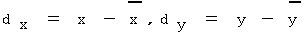Let sx, sy be the standard deviations of X, Y

Let r be the correlation coefficient of X, Y (in modern notation, this is the population correlation r)

Let z be the frequency of a pair of values (x, y), such that x falls between [dx, dx + dx], and y between [dy, dy + dy]. Pearson's preceding work determined the following expression for z:Note, that in modern terms, z/N is exactly the probability density function of the bivariate normal distribution. Multiplying the p.d.f. by the number of observations, N, gives the theoretical frequency of values within a d-neighborhood about (x,y)(11).

Consider n pairs of organs (x1,y2) ... (xn,yn), then the probability this sequence occurs is dependent upon:Now, since sx,sy are the mean deviations from the respective means of X & Y , substitute them into the expression: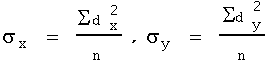Let l = (Sdxdy) / (nsxsy), then,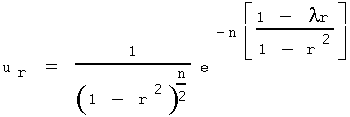Or, in a more compact form: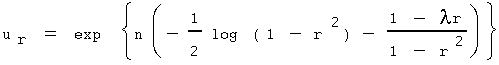Pearson's goal is to maximize this function. Note that ur attains a maximum whenever the expression in parentheses attains a maximum. Pearson expands ur through Taylor's Theorem(12) to examine the coefficients, which are the derivatives of ur. Suppose a new value of r(13), say r', such that r r'. Remember that r is equivalent to the modern r, and although Pearson did not state it this way, he is seeking a formula that will provide an estimate of r. Pearson expands the expression,by applying Taylor's Theorem for approximating a function near a point. The point chosen is r, the true correlation value for which Pearson seeks to derive a best estimate. The first few terms of the Taylor expansion are:Simplifying,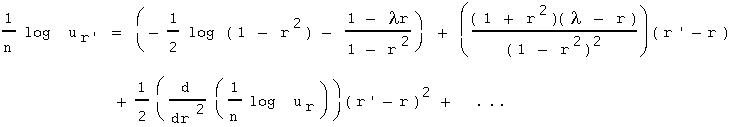Here(14),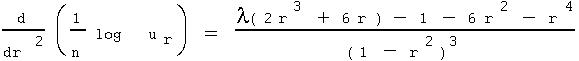Clearly, the first derivative term (r' - r) will be zero when r = l. So r = l is a critical point(15). When r = l the coefficient of the (r' - r)2 term, which is the second derivative, becomes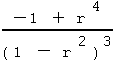and is clearly negative (recall -1 < r < 1), and therefore r = l is a maximum. Therefore, the best value of r (i.e. the maximum likelihood estimator) is obtained by:Outline of the Derivation of the Probability of Chi-squared:

adapted from Karl Pearson's "On the Criterion that a given System of Deviations from the Probable in the case of a Correlated System of Variables is such that it can be reasonably supposed to have arisen from Random Sampling" published in 1900.

A system of n deviations(16) in frequency from their mean (expected) values is described by c2 = the equation of a generalized ellipsoid in n-space. The c2 = constant is a particular system of deviations, and therefore let c2 = c for the set of observed deviations. Pearson then applies the frequency definition of probability: P = the number of points in the event space divided by the number of points in the space of all possible events. This translates into integration when the number of points is no longer finite. To determine the probability of the observed system of deviations and all more deviant (and less likely) such systems, Pearson calculates: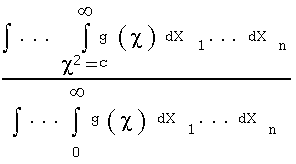Pearson divides the n-fold integral of a function, which I have denoted g()(17), from c to infinity by the n-fold integral from 0 to infinity. Here X1 ... Xn are orthogonal basis vectors in n-space. By applying a transform of variables, these integrals can be reduced by integration by parts to an expression used to determine the probability of a computed c2 statistic.

Notes:

1. A test of homogeneity examines the degree to which "two (or more) distributions exhibit the same profile of frequencies across two or more categories." Categories may represent "numerical values, numerical intervals, or nominal classes." (Frank 635).
2. Also known as the phi coefficient for 2x2 tables (Frank 664).
3. This is a faulty statistical argument; correlation does not imply a cause-effect relationship. However, it is difficult to argue for a cause-effect relationship if the two variables are uncorrelated.
4. However, Galton himself never dealt with negative correlation coefficients.
5. By organ, Galton refers to the arm, leg, head, etc.
6. As conceived, the correlation coefficient only applied to data which followed the normal law.
7. Formula when the mean is estimated (unknown), with N-1 in the denominator instead of N.
8. At least this is true for tests of independence and homogeneity; I am uncertain about other contingency table tests involving Chi-squared.
9. Exact: this test is not just an approximation based upon asymptotic results.
10. Pearson used x,y to represent the deviations, but this would be easily confused with modern notation.
11. Recall that for a continuous distribution, the probability at a single point is always zero.
12. I am not certain why Pearson doesn't simply compute the first two deratives and reach his conclusion, instead of expanding by Taylor's Theorem, perhaps it's related to the mathematical methods of the times.
13. Actually, Pearson wrote: "assume r to differ by r from the value previously selected." However, this does not match the modern notation for r.
14. Here, I have not confirmed Pearson's calculation of the coefficient of the (r' - r)2 term, although I did so for the (r '- r) term.
15. This is so clear that Pearson doesn't even feel he needs to mention it.
16. Pearson assumes these deviations are from a multivariate Normal distribution; the theory is more widely applicable because of convergence theorems.
17. This function is the exponential kernel of the Normal distribution.
Sources:

Barnard, G. A. "Introduction to Pearson (1900) On the Criterion that a Given System of Deviations in the Case of a Correlated System of Variables is Such that is Can be Reasonably Supposed to have Arisen from Random Sampling." In Breakthroughs in Statistics, Volume II (S. Kotz and N. L. Johnson, eds). New York: Springer-Verlag, 1992. pg 1 - 10.

Box, Joan Fisher. R. A. Fisher: The Life of a Scientist. New York: John Wiley & Sons, 1978.

Frank, Harry & Althoen, Steven C. Statistics: Concepts and Applications. New York: Cambridge University Press, 1994. chaps 4, 14, 15.

Galton, Francis. "Co-relations and their Measurement, chiefly from Anthropometric Data." Proceedings of the Royal Society of London, v45 (Nov 15 - April 11) 1888.

Pearson, Egon Sharpe. 'Student': A Statistical Biography of William Sealy Gosset. Oxford: Clarendon Press, 1990. pg. 23 - 44.

Pearson, Karl. "Mathematical Contributions to the Theory of Evolution III. Regression, Heredity, and Panmixia." (1896) In Karl Pearson's Early Statistical Papers. London: Cambridge University Press, 1956. pg. 113 - 178.

Pearson, Karl. "On the Criterion that a given System of Deviations from the Probable in the case of a Correlated System of Variables is such that it can be reasonably supposed to have arisen from Random Sampling." (1900) In Karl Pearson's Early Statistical Papers. London: Cambridge University Press, 1956. pg. 339-357.

Rinaman, William C. Foundations of Probability and Statistics. San Diego: Saunders College Publishing, 1993. chaps 5, 13.

Stewart, James. Calculus: Early Transcendentals. Pacific Grove, CA: Brooks/Cole Publishing, 1991. pg. 602 - 625.

Tankard, James W, Jr. The Statistical Pioneers. Cambridge, MA: Schenkman Publishing Co, Inc., 1984.

Weldon, W. F. R. "Certain Correlated Variations in Crangon vulgaris." Proceedings of the Royal Society of London, v51 n314, (Mar 3 - May 19) 1892.